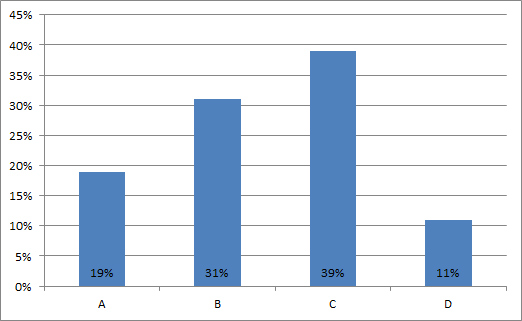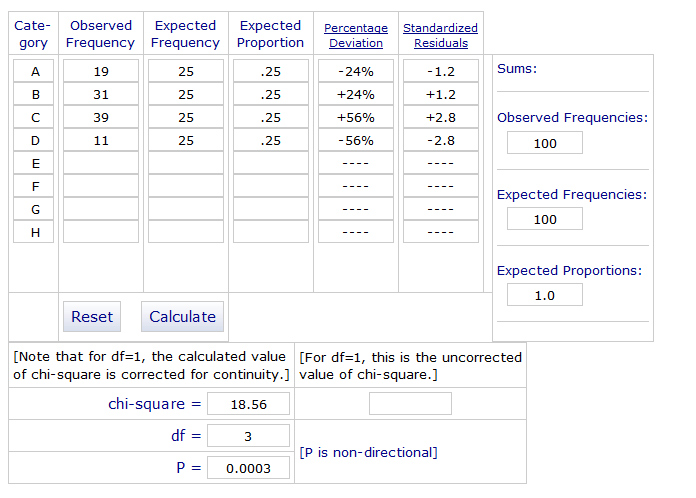# How to Statistically Test Preference Data

Jeff Sauro, PhDWhich design do you prefer?

Which product would you pick?

Which website do you find more usable?

A cornerstone of customer research is asking preferences.

A common scenario is to show participants a set of designs or two or more websites, and then ask customers which design or site they prefer. While customer preferences often don’t match customer performance, it’s good practice to collect both and then reconcile differences.

While it’s easy to ask a preference question, it’s a bit less straightforward to analyze because there are multiple approaches.

There are actually a number of reasonable ways to analyze preferences though (binomial test, confidence interval test, Chi-Square Goodness of Fit test and McNemar Exact test). I’ll start with the approach I use and recommend: the binomial test with confidence intervals.

## Binomial Test with a Confidence Interval

Let’s start with an example. You present 100 participants with four designs and ask them which one they prefer. Figure 1 below shows the results in a graph. Design C was the most selected with 39 out of 100 participants choosing it. But how do you know if the choice of Design C is statistically significant?Figure 1: Percentage of choices 100 participants made. Design C was chosen the most, but is it statistically significant?

To answer this question, start with what you’d expect from chance. If participants were randomly picking, or really didn’t have much of an opinion, you would expect 25% to pick any given design. But randomness is lumpy; any choice near 25% is hard to differentiate from random selection, so you need the selection to be sufficiently different than 25%. Design C is certainly above 25% and Designs A and D are below 25%, but are they different enough?

To differentiate true choice from random chance, you can use the one-sample binomial test with a confidence interval. You can follow along using the online calculator.Figure 2: Screenshot of the One Sample Proportion Calculator to perform the binomial test.

1. Start with the design that had the highest percentage of the vote (Design C with 39%).
2. Enter the number of participants that selected the design (39) and the total number in the study (100).
3. Divide the number of choices into 1 to find the test proportion. In this example, you have 4 choices, so the test proportion is .25. (1/4 =.25). If you had 3, it’d be 1/3 = .333.
4. Enter the test proportion of .25 and select “Is Not Equal to.”
5. Click Submit to get the results. The p-value is .0024. Because this is low (less than .05) you can conclude Design C is statistically significant. That is, it’s unusual to see such a high percentage for a choice if participants really didn’t have a preference.

You can also estimate the percentage of all customers that may choose this design by using a confidence interval, which is also included in the results. The 95% confidence interval is 30% to 49%. The lower boundary of the confidence interval tells you the preference is unlikely to dip below 30%, which is still above the chance threshold of 25%. So you know the choice of Design C is statistically significant and the winning design.

### Chi-Square Goodness of Fit test

An alternative approach to a binomial test with confidence intervals is to use the Chi-Square Goodness of Fit test. By testing the observed distribution (19%, 31%, 39%, 11%) against the expected distribution ( 25%, 25%, 25%, 25%), you can see how much the distribution differs from chance. Figure 3 below shows a screenshot from an online calculator.Figure 3: Screenshot of a Chi-Square Goodness of Fit Calculator.

The Chi-Square statistic in this example also generates a statistically significant p-value (p = .0003) but it doesn’t tell you if a design is winning, only whether the distribution differs from the expected one. So it’s less clear whether Design C is deviating from 25% (because it’s selected more often) or whether it’s Design D and A (because they are selected least).

## The Confidence Interval Method

Another services to determine whether there are differences between designs is to compute confidence intervals around each choice. Figure 4 below shows the 95% confidence intervals and the table shows the values.Figure 4: Percentage of choices 100 participants made with 95% confidence intervals.

 Design Choice CI Low CI High A 19% 12% 28% B 31% 23% 41% C 39% 30% 49% D 11% 6% 19%

You can tell two things with the confidence interval method:

• Only Design C has a confidence interval lower boundary that is higher than 25%, which is the threshold for chance. While Design B was selected by 31% of the participants, you can only be 95% confident at least 23% would select it, so you can’t differentiate that selection from chance.
• The overlap in the confidence intervals determines whether design preferences are statistically different from each other. While Design C was selected the most, it’s not statistically different than Design B. The error bars on the graph overlap. In contrast, the error bars for Design C don’t overlap with Designs A and D, meaning more people would select C than A and D.

The confidence interval approach is more conservative than the one-sample binomial test and Chi-Square test. The reason is that it doesn’t take into account the within-subjects nature of a preference task. That is, the choices are dependent, if participants pick C, they can’t pick A, B, or D. If you fail to detect a statistical difference with a confidence interval approach, you may want to use the binomial test.

The upside to a confidence interval though is if you do detect a statistical difference, you can have more confidence the difference isn’t due to chance because it’s more conservative than the binomial test.

### The McNemar Exact test

The McNemar Exact test takes into account the dependency between selections and allows you to compare each combination of designs (e.g. B compared to C) and not be as conservative as the confidence interval approach. One drawback is that you need the original raw data (and not the summary percentages) so it takes more effort to compute. See Chapter 5 in Quantifying the User Experience to find out how to use the McNemar Exact test.

## Summary

When testing preference data, use the following approaches:

• Compare the most selected choice using the one-sample binomial against random chance (5 choices = .20, 4 choices = .25, 3 choices = .333 and 2 choices = .5).
• An alternative is the Chi-Square Goodness of Fit test to see whether the distribution deviates from chance. Significant p-values won’t tell you whether the deviation is for the most or least selected choice. Compute a confidence interval to compare the alternatives directly. Keep in mind it’s more conservative because a confidence interval doesn’t take into account the dependencies between choices.
• To compare choices directly and take into account dependence, use the McNemar Exact test.
0
0